# 写在最前面

「有研究显示，打字的时候不喜欢在中文和英文之间加空格的人，感情路都走得很辛苦，有七成的比例会在 34 岁的时候跟自己不爱的人结婚，而其余三成的人最后只能把遗产留给自己的猫。毕竟爱情跟书写都需要适时地留白。

# 绪论

## 基本术语

### 术语例题

1 张三 程序员
2 李四 企业家
3 王五 公务员
4 周六 学生
5 钱七 教师
• 上表中样本是？
• 一共有多少样本？
• 样本的属性都是？
• 样本标记是？
• 用户「周六」属性值和标记值是？

• 样本是顾客。
• 一共 5 个。
• 年收入、性别和职业。
• 是否为顾客（或好顾客）。
• 属性值&标记值：年收入=低、性别=女和职业=学生。

## 假设空间

1 青绿 蜷缩 浊响
2 乌黑 蜷缩 浊响
3 青绿 硬挺 清脆
4 乌黑 稍蜷 沉闷

### 版本空间例题1

1 张三 程序员
2 李四 企业家
3 王五 公务员
4 周六 学生
5 钱七 教师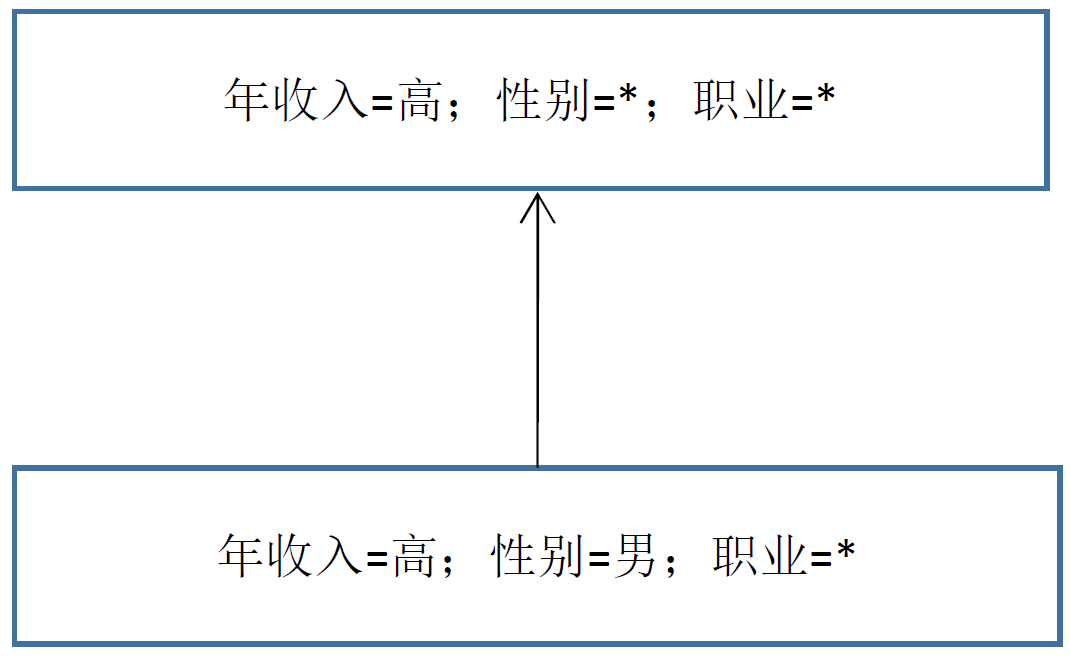### 版本空间例题2

1 青绿 蜷缩 浊响
2 乌黑 蜷缩 浊响
3 青绿 硬挺 清脆
4 乌黑 稍蜷 沉闷# 归纳偏好

## 奥卡姆剃刀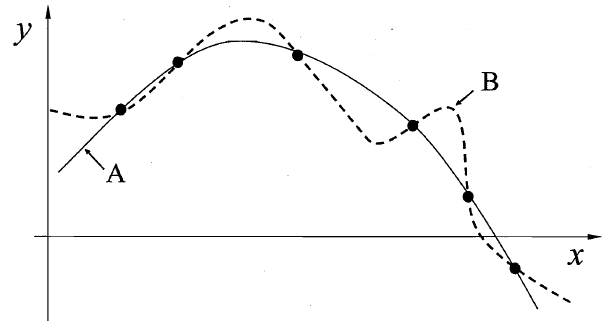# 经验误差与过拟合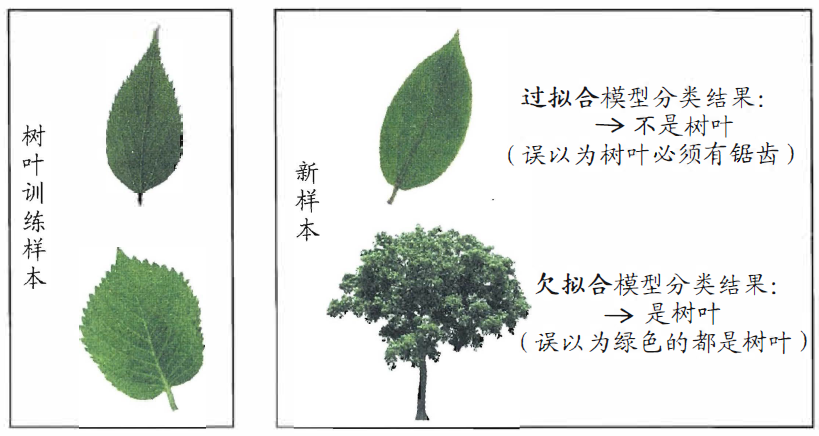## 交叉检验法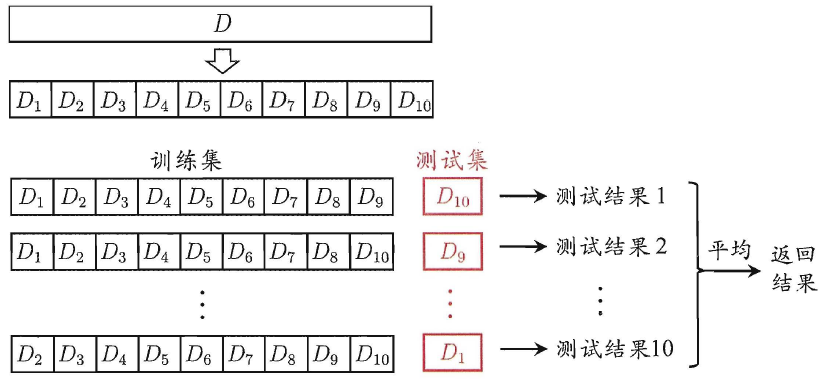## 自助法

\begin{aligned} P&=\lim\limits_{m→∞}(1-\frac{1}{m})^m \\ &=\frac{1}{e} \\ &≈0.368 \end{aligned}

# 性能度量

$E(f;D) = \frac{1}{m}\sum_{i = 1}^m (f(x_i) - y_i)^2$

$E$：数学期望，也就是均值。是一种概率论概念，样本出现的情况结合出现的概率，是一种加权平均。（最后一步都是求平均值，这个公式其实和平均值有点类似）

$f$：代指我们训练的模型。

$D$：数据集。

$m$：样本的总数量。

$i$：当前样本的下标。

$x_i$：第 i 个样本的预测结果的标签（好瓜/坏瓜）。

$y_i$：第 i 个样本的标签真实值。

$\displaystyle\sum_{i=1}^m(x_i)$：通过下标将所有 x 相加起来。和 for 循环差不多。

## 错误率与精度

### 错误率

$E(f;D) = \frac{1}{m}\sum_{i = 1}^m \text{Ⅱ}(f(x_i) \ne y_i)$

$E(f;D)$：对模型 $f$ 和数据集 $D$ 的数学期望。

$m$：样本的总数量。

$i$：当前样本的下标。

$x_i$：第 i 个样本的预测结果的标签（好瓜/坏瓜）。

$y_i$：第 i 个样本的标签真实值。

$Ⅱ$：相当于定义的一个函数，和 $f(x)$ 概念类似，只是它这个符号比较迷惑。

$Ⅱ(f({x_i}) \ne {y_i}) = \begin{cases} 1 ,&\text{if }f(x_i) \ne y_i \\ 0 ,&\text{if }f(x_i) = y_i \end{cases}$

• 如果预测值和实际值相等，那么这个函数输出 0。
• 如果预测值和实际值有偏差，那么这个函数输出 1。

### 精度

$acc(f;D) = 1-E(f;D)$

$acc(f;D)$：acc 是 Accuracy 的缩写，意思是 准确/精确 率。

$E(f;D)$：就是上面的错误率。

$E(f;D) = \frac{1}{m}\sum\limits_{i = 1}^m Ⅱ(f({x_i}) \color{blue}= \color{black}{y_i})$

$Ⅱ$ 函数改成：

• 如果预测值和实际值相等，那么这个函数输出 1。
• 如果预测值和实际值有偏差，那么这个函数输出 0。

$Ⅱ(f({x_i}) \color{blue}=\color{black} {y_i}) = \begin{cases} 1 ,&\text{if }f(x_i) \color{blue}=\color{black} y_i \\ 0 ,&\text{if }f(x_i) \color{blue}\ne\color{black} y_i \end{cases}$

## 查准率与查全率

$p=\frac{TP}{TP+FP}$

$R=\frac{TP}{TP+FN}$

## F1 度量

F1 度量是用于找到一个查全率和查准率的平衡点算法。

$F1 = \frac{2×P×R}{P+R} = \frac{2×TP}{样例总数+TP-TN}$

F1 度量还可以通过调整参数，让他向查全率偏重，或者是向查准率偏重。

$F_β = \frac{(1+β^2)×P×R}{(β^2×P)+R}$

$F_β = \begin{cases} 偏重查全率 &β>1\\ 标准 F1 &β=1\\ 偏重查准率 &β<1 \end{cases}$

## 宏/微F1

• 宏：先计算查准率和查全率再求平均值。
• 微：先计算 TP、FP、TN、FN 的平均值再求查准率和查全率。

### 宏

$P=\frac{TP}{TP+FP}$

$R=\frac{TP}{TP+FN}$

$macro-P = \frac{1}{n}\sum_{i=1}^nP_i$

$macro-R = \frac{1}{n}\sum_{i=1}^nR_i$

$F1 = \frac{2×P×R}{P+R}$

$macro-F1 = \frac{2×macro-P×macro-R}{macro-P+macro-R}$

### 微

$TP$ 的平均值为 $\overline{TP}$

$FN$ 的平均值为 $\overline{FN}$

$FP$ 的平均值为 $\overline{FP}$

$TN$ 的平均值为 $\overline{TN}$

$P=\frac{TP}{TP+FP}$

$R=\frac{TP}{TP+FN}$

$micro-P = \frac{\overline{TP}}{\overline{TP}+\overline{FP}}$

$micro-R = \frac{\overline{TP}}{\overline{TP}+\overline{FN}}$

$micro-F1 = \frac{2×micro-P×micro-R}{micro-P×micro-R}$

## P-R ROC AUC

### P-R

P-R 曲线横坐标是查全率：

$R=\frac{TP}{TP+FN}$

$P=\frac{TP}{TP+FP}$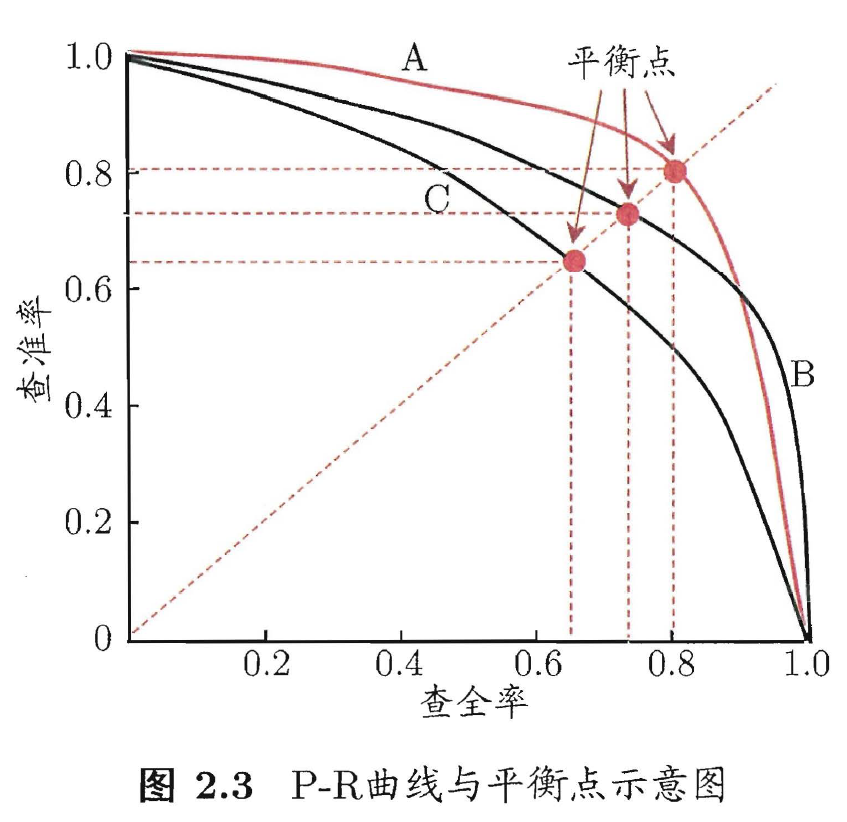### ROC

ROC 曲线的横坐标是假正例率（Flase Positive Rate）：

$FPR=\frac{FP}{FP+TN}$

$TPR=\frac{TP}{TP+FN}$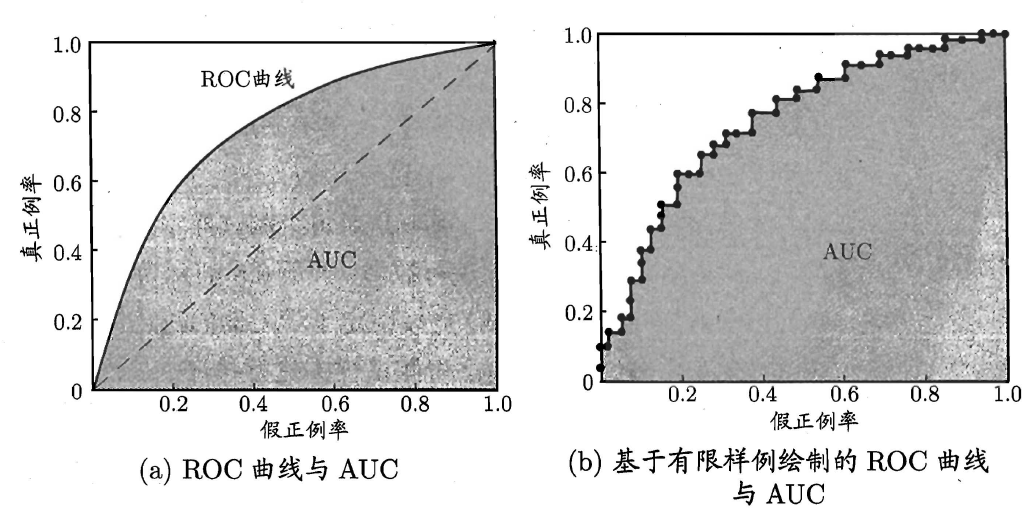### AUC

AUC（Area Under ROC Curve）指的 ROC 曲线下面的面积，而这个面积越大，这个模型就越好。

cluster 每个组就是一个簇

# 参考

• 本文作者： 蠢黑通行
• 本文链接： https://blackyau.cc/26.html
• 版权声明： 本博客所有文章除特别声明外，均采用 BY-SA 许可协议。转载请注明出处！